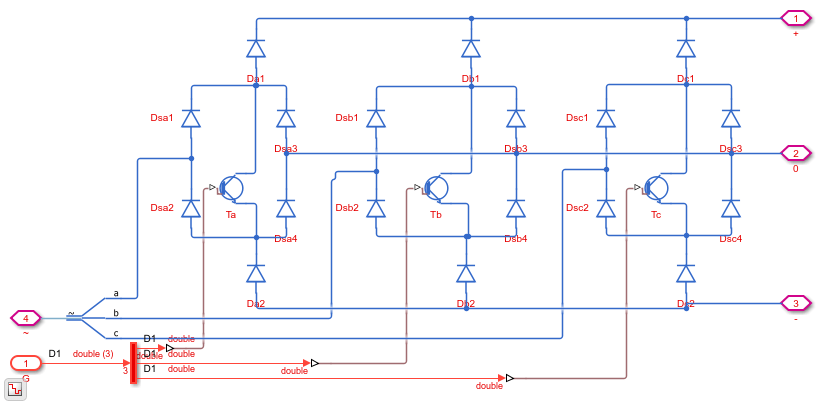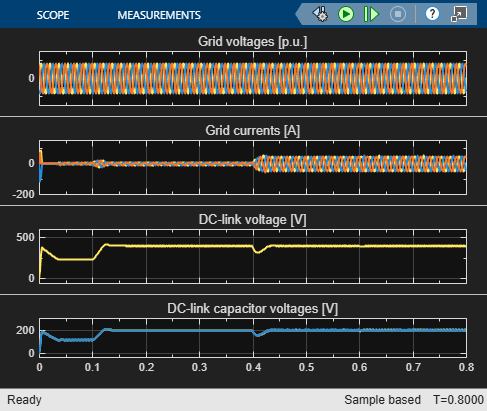# Generate Optimized HDL Implementation Model from Simscape

This example shows how you can generate an optimized HDL implementation model for a Simscape™ vienna rectifier model by using optimizations such as resource sharing and RAM mapping.

### Why Optimize the HDL Implementation Model

For Simscape models that have many switching elements, the state-space representation contains a large number of configurations. The Simscape HDL Workflow Advisor simulates the Simscape model to calculate the number of relevant configurations. Certain Simscape models can have a large number of configurations that are relevant. The generated HDL implementation model for such a large design can consume a significantly large number of resources. Synthesizing the generated code can cause the design to occupy a large amount of resources on the FPGA device. In some cases, the design might not fit on the target FPGA device. To save resoures and make the design fit on the FPGA, the Simscape HDL Workflow Advisor uses HDL Coder™ optimizations such as clock-rate pipelining, resource sharing, and RAM mapping.

### Vienna Rectifier Model

To open the model, at the MATLAB® command prompt, enter:

```open_system('sschdlexViennaRectifierExample') ```

Save this model as ViennaRectifier_HDL to run the workflow.

```open_system('ViennaRectifier_HDL') set_param('ViennaRectifier_HDL', 'SimulationCommand', 'Update') ```The `Control` subsystem implements a closed-loop control strategy for the `Vienna rectifier` subsystem by using space-vector modulation. At simulation time `0.1s`, the vienna rectifier is engaged. At times `0.4s` and `0.6s`, the load steps up on the DC side.

The `Vienna rectifier` subsystem consists of three-phase legs. Each leg has one power switch and six power diodes. See Vienna Rectifier Control (Simscape Electrical).

```open_system('ViennaRectifier_HDL/Vienna rectifier') ```Simulate the model. View the simulation results by double-clicking the Scope blocks inside the `Scopes` subsystem.

```sim('ViennaRectifier_HDL') open_system('ViennaRectifier_HDL/Scopes/Scope') ```### Generate HDL Implementation Model and Validate HDL Algrithm

To generate an HDL implementation model, use the Simscape HDL Workflow Advisor. You can generate HDL code for the implementation model. To open the Advisor, run this command:

```sschdladvisor('ViennaRectifier_HDL') ```

To run the workflow, right-click the Generate implementation model task and select Run to Selected Task. After the task passes, you see a link to the HDL implementation model. To see the number of configurations, select the Extract Equations task. On the task, you see that simulating the model reaches `558` modes. Such a large number of modes can increase resource consumption of the design on the FPGA.

To open the HDL implementation model, enter these commands:

```open_system('gmStateSpaceHDL_ViennaRectifier_HDL') set_param('gmStateSpaceHDL_ViennaRectifier_HDL', 'SimulationCommand', 'Update') ```The ports of this subsystem use the same name as the Simulink-PS Converter and PS-Simulink Converter blocks in your original Simscape model. If you navigate inside this subsystem, you see several delays, adders, and Matrix Multiply blocks that model the state-space equations.

```open_system('gmStateSpaceHDL_ViennaRectifier_HDL/HDL Subsystem/HDL Algorithm') ```To validate the HDL algorithm, in the Generate implementation model task, select the Generate validation logic for the implementation model check box, set the Validation logic tolerance to `0.001`, and rerun this task. The task generates a state-space validation model that compares the implementation model and the original Simscape model.

```open_system('gmStateSpaceHDL_ViennaRectifier_HDL_vnl') set_param('gmStateSpaceHDL_ViennaRectifier_HDL_vnl', 'SimulationCommand', 'Update') ```Simulating the model does not display assertions, which indicates that the HDL algorithm matches the original model.

```sim('gmStateSpaceHDL_ViennaRectifier_HDL_vnl') ```

### Map State-Space Parameters in Implementation Model to RAM

The HDL implementation model uses `single` data types and contains large Delay blocks that are inside a feedback loop in the `HDL Algorithm` subsystem. To accommodate the large delays and make the design run at a faster clock rate on the target FPGA, the model uses clock-rate pipelining in conjunction with a large value of Oversampling factor.

```hdlsaveparams('gmStateSpaceHDL_ViennaRectifier_HDL') ```

In the Generate implementation model task, the Map state space parameters to RAMs setting uses the default value of `Auto`. This setting maps large state-space parameters in the HDL implementation model to RAMs when the number of modes exceed a threshold value of `200`. As the vienna rectifier model uses a large number of modes, the state-space parameters are mapped to RAMs. By mapping to RAMs, you save lookup table resources on the FPGA. To enable the RAM mapping, the UseRAM parameter is enabled on the masked subsystem blocks that perform the state update and compute the output.

To map the parameters to RAMs irrespective of the threshold, set Map state space parameters to RAMs to `on`.

To see the effect of RAM mapping on the vienna rectifier model:

1. Verify the UseRAM parameter setting by running the `hdlget_param` function on the `Multiply Input` and `Multiply State` blocks.

```Multiplysubsys1 = 'gmStateSpaceHDL_ViennaRectifier_HDL/HDL Subsystem/HDL Algorithm/State Update'; Multiplysubsys2 = 'gmStateSpaceHDL_ViennaRectifier_HDL/HDL Subsystem/HDL Algorithm/Output'; UseRAM1 = hdlget_param([Multiplysubsys1 '/Multiply Input'], 'UseRAM') UseRAM2 = hdlget_param([Multiplysubsys1 '/Multiply State'], 'UseRAM') ```
```UseRAM1 = 'on' UseRAM2 = 'on' ```

2. Enable generation of the resource utilization report.

```hdlset_param('gmStateSpaceHDL_ViennaRectifier_HDL', 'ResourceReport', 'on') ```

3. Generate HDL code for the implementation model.

```makehdl('gmStateSpaceHDL_ViennaRectifier_HDL/HDL Subsystem'); ```

When you generate code, HDL Coder opens a Code Generation report. The High-level Resource Report shows `136` RAMs utilized.### Resource Sharing of State Update and Output Computation Blocks

Before you generate HDL code for the HDL Subsystem, you can optimize the algorithm by using the resource sharing optimization in HDL Coder. Resource sharing is an area optimization that identifies multiple functionally equivalent resources and replaces them with a single, equivalent resource. The data is time-multiplexed over the shared resource to perform the same operations. See Resource Sharing.

In the HDL implementation model, you can share the masked subsystem blocks that perform state updates and compute the output.

To share these subsystems for the vienna rectifier and generate HDL code:

1. Specify a SharingFactor of 2 on the Multiply Input and Multiply State subsystems.

```hdlset_param([Multiplysubsys1 '/Multiply Input'],'SharingFactor', 2) hdlset_param([Multiplysubsys1 '/Multiply State'],'SharingFactor', 2) hdlset_param([Multiplysubsys2 '/Multiply Input'],'SharingFactor', 2) hdlset_param([Multiplysubsys2 '/Multiply State'],'SharingFactor', 2) ```

2. Enable generation of the optimization report

```hdlset_param('gmStateSpaceHDL_ViennaRectifier_HDL', 'OptimizationReport', 'on') ```

3. Generate HDL code for the HDL Subsystem block in the implementation model.

```makehdl('gmStateSpaceHDL_ViennaRectifier_HDL/HDL Subsystem'); ```

When you generate code, HDL Coder opens a Code Generation report. To see the status of the resource sharing optimization, click the Streaming and Sharing section of the report. This sharing group shows the dot products that the optimization shared. When you click the High-level Resource Report, you see that the comsumption of adders, multipliers, and registers have decreased.